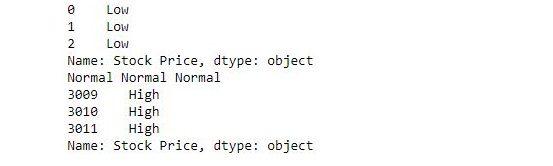Open In App
Related Articles
• Write an Interview Experience
• Pandas Tutorial

# Python | Pandas.apply()

Pandas.apply allow the users to pass a function and apply it on every single value of the Pandas series. It comes as a huge improvement for the pandas library as this function helps to segregate data according to the conditions required due to which it is efficiently used in data science and machine learning.

Installation:
Import the Pandas module into the python file using the following commands on the terminal:

```pip install pandas
```

To read the csv file and squeezing it into a pandas series following commands are used:

```import pandas as pd
```

Syntax:

`s.apply(func, convert_dtype=True, args=())`

Parameters:

func: .apply takes a function and applies it to all values of pandas series.
convert_dtype: Convert dtype as per the function’s operation.
Return Type: Pandas Series after applied function/operation.

Example #1:

The following example passes a function and checks the value of each element in series and returns low, normal or High accordingly.

 `import` `pandas as pd`` ` `# reading csv``s ``=` `pd.read_csv(``"stock.csv"``, squeeze ``=` `True``)`` ` `# defining function to check price``def` `fun(num):`` ` `    ``if` `num<``200``:``        ``return` `"Low"`` ` `    ``elif` `num>``=` `200` `and` `num<``400``:``        ``return` `"Normal"`` ` `    ``else``:``        ``return` `"High"`` ` `# passing function to apply and storing returned series in new``new ``=` `s.``apply``(fun)`` ` `# printing first 3 element``print``(new.head(``3``))`` ` `# printing elements somewhere near the middle of series``print``(new[``1400``], new[``1500``], new[``1600``])`` ` `# printing last 3 elements``print``(new.tail(``3``))`

Output:Example #2:

In the following example, a temporary anonymous function is made in .apply itself using lambda. It adds 5 to each value in series and returns a new series.

 `import` `pandas as pd``s ``=` `pd.read_csv(``"stock.csv"``, squeeze ``=` `True``)`` ` `# adding 5 to each value``new ``=` `s.``apply``(``lambda` `num : num ``+` `5``)`` ` `# printing first 5 elements of old and new series``print``(s.head(), ``'\n'``, new.head())`` ` `# printing last 5 elements of old and new series``print``(``'\n\n'``, s.tail(), ``'\n'``, new.tail())`

Output:

```0    50.12
1    54.10
2    54.65
3    52.38
4    52.95
Name: Stock Price, dtype: float64

0    55.12
1    59.10
2    59.65
3    57.38
4    57.95
Name: Stock Price, dtype: float64

3007    772.88
3008    771.07
3009    773.18
3010    771.61
3011    782.22
Name: Stock Price, dtype: float64

3007    777.88
3008    776.07
3009    778.18
3010    776.61
3011    787.22
Name: Stock Price, dtype: float64
```

As observed, New values = old values + 5